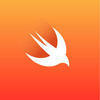## Welcome to the Treehouse Community

The Treehouse Community is a meeting place for developers, designers, and programmers of all backgrounds and skill levels to get support. Collaborate here on code errors or bugs that you need feedback on, or asking for an extra set of eyes on your latest project. Join thousands of Treehouse students and alumni in the community today. (Note: Only Treehouse students can comment or ask questions, but non-students are welcome to browse our conversations.)

### Looking to learn something new?

Treehouse offers a seven day free trial for new students. Get access to thousands of hours of content and a supportive community. Start your free trial today.

# What is wrong with this code?

```func temperatureInFahrenheit(temperature: Double)-> Double {
result = (temperature * 9) / 5
result = result + 32
return result
}
```

Hi there,

In your code, `result` isn't declared. Add the word `var` before its first usage - that will fix your code.

```func temperatureInFahrenheit (temperature: Double) -> Double {
var result = (temperature * 9) / 5
result = result + 32
return result
}
```

Alternatively, try a solution on one line like this:

```func temperatureInFahrenheit(temperature: Double) -> Double {
return ((temperature * 9) / 5) + 32
}
```

I hope that helps.

Steve.Hey Abdijabar Mohamed,

The code challenge asks us to create a function that converts a temperature from Celsius to Fahrenheit. The problem with your code is that you're not declaring result as a variable or a constant. To get your code to pass, you need to declare result as a variable.

```func temperatureInFahrenheit(temperature: Double) -> Double {
var result = (temperature * 9) / 5
result = result + 32
return result
}
```

You can condense the operations of the function to be on a single line, because of the order of operations. Here's the solution I recommend:

```func temperatureInFahrenheit(temperature: Double) -> Double {
let result = (temperature * 9)/5 + 32
return result
}
```

Good Luck!

Thanks a heap

Thanks a heap